﻿ 利用重庆地震台网P波记录测定<i>M</i><sub>WP</sub>震级文章快速检索
 地震地磁观测与研究2018, Vol. 39Issue (4): 12-17  DOI: 10.3969/j.issn.1003-3246.2018.04.0020

引用本文Chen Lijuan, Gong Liwen, Wang Tongjun. MWP magnitude calculated with P wave records in Chongqing Seismic Network[J]. Seismological and Geomagnetic Observation and Research, 2018, 39(4): 12-17. DOI: 10.3969/j.issn.1003-3246.2018.04.002.

文章历史

MWP magnitude calculated with P wave records in Chongqing Seismic Network
Chen Lijuan, Gong Liwen, Wang Tongjun
Chongqing Earthquake Agency, Chongqing 401147, China
Abstract: Using the earthquakes with MS > 3.0 recorded in Chongqing Seismic Network since 2010 to 2016, the amplitude and cycle information, namely the long-period spectral level and the moment and the corner cycle of P wave are gotten through spectrum analysis. The relation between the corner period of P wave and the MS, and the relation between the long-period spectral level of P wave and the MS are obtained. The MWP is calculated through the P wave moment. The difference between MWP and MS is analyzed. The results show that the MWP is larger than MS when MS ≤ 4.0, and the MWP is smaller than MS when MS > 4.0. The results of hypothesis testing show that the MWP and the magnitude calculated from the long-period spectral level of P wave is relatively close while the magnitude calculated from the P wave corner cycle is in large difference.
Key words: corner period of P wave    long-period spectral level of P wave    moment magnitude of P wave
0 引言

1 数据资料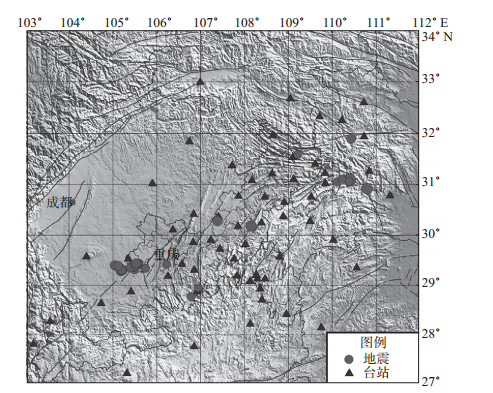图 1 地震震中及台站分布 Fig.1 The distribution of epicenters and stations
2 方法步骤

 $\mathit{\Omega }\left(\mathit{f} \right) = \frac{{{\mathit{\Omega }_{\rm{0}}}}}{{1 + {{\left({\mathit{f}{\rm{/}}{\mathit{f}_{\rm{c}}}} \right)}^2}}}$ (1)

 ${\mathit{\tau }_{{\rm{cP}}}}{\rm{ = 1/}}{\mathit{f}_{{\rm{cP}}}}$ (2)
 ${\mathit{M}_{{\rm{0p}}}} = \frac{{4\pi \mathit{\rho }{\mathit{\alpha }^{\rm{3}}}{\mathit{\Omega }_{{\rm{0p}}}}\mathit{d}}}{\mathit{R}}$ (3)

 ${\mathit{M}_{{\rm{WP}}}}{\rm{(lg}}{\mathit{M}_{{\rm{0P}}}}{\rm{ - 9}}{\rm{.1) /1}}{\rm{.5}}$ (4)

3 计算结果表 1 所选地震P波计算结果及震级对比 Tab.1 The results obtained from P waves of selected earthquake and the comparison of magnitude

(1) MSMWP震级大小对比。将MS与P波矩震级MWP进行对比分析，按照MS递增排序，得到MSMWP关系曲线，见图 2。由图 2可见，当MS≤4.0(图中1—27号地震)时，MWPMS；当MS＞4.0(图中28—33号地震)时，MWPMS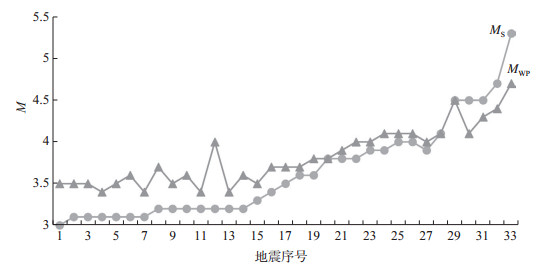图 2 MS与MWP关系对比 Fig.2 The comparison of MS and MWP

(2) MWPMS差值统计。33次地震的P波矩震级MWPMS差值统计关系见图 3。由图 3可见，MWPMS的差值主要在0—0.5范围内，呈近似正态分布。MWP-MS = 0.2的地震数最多，差值0.1地震次数次之，说明参与计算的大部分地震的P波矩震级MWPMS相差较小。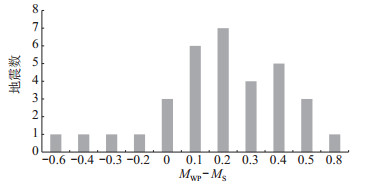图 3 MWP与MS震级差统计 Fig.3 The statistics of magnitude difference between MWP and MS表 2 不同震级区间震级差统计 Tab.2 The statistics of magnitude difference in different magnitude range

(3) MSMWP震级关系式。通过对比，计算P波矩震级MWP与重庆地震台网MS的关系式，如图 4所示，除个别地震差别较大外，P波矩震级MWPMS表现为相关性较好的线性关系。拟合得到以下关系式

 ${\mathit{M}_{\rm{S}}}{\rm{ = 1}}{\rm{.5958}}{\mathit{M}_{{\rm{WP}}}}{\rm{ - 2}}{\rm{.4738}}$ (5)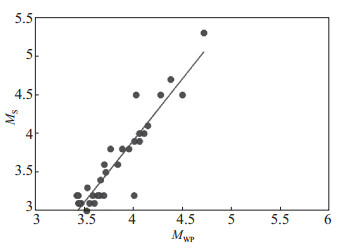图 4 MWP与MS的关系 Fig.4 The relation between MWP and MS

(4) Ω0PMS的拟合关系。图 5为P波零频极限值Ω0P与重庆地震台网MS的统计关系，可见二者相关性较好。当计算得到P波的零频极限值Ω0P时，可由以下拟合关系式计算MS

 ${\mathit{M}_{\rm{S}}}{\rm{ = 1}}{\rm{.0638lg}}{\mathit{\Omega }_{{\rm{0P}}}}{\rm{ - 5}}{\rm{.0531}}$ (6)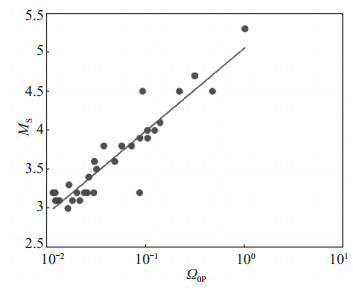图 5 Ω0P与MS的关系 Fig.5 The relation between Ω0P and MS

(5) τcPMS的拟合关系。图 6给出P波拐角周期τcP与震级MS之间的拟合关系，可见τcP呈现出随震级增加而增加的趋势，但二者相关性较差，主要原因可能是拐角频率的测量误差较大。二者拟合关系式如下

 ${\mathit{M}_{\rm{S}}}{\rm{ = 1}}{\rm{.7718}}{\mathit{\tau }_{{\rm{cP}}}}{\rm{ - 2}}{\rm{.6822}}$ (7)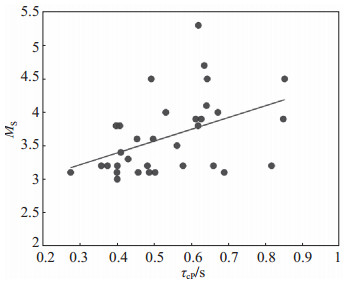图 6 τcP与MS的关系 Fig.6 The relation between τcP and MS
4 结果检验表 3 2017年地震检验结果 Tab.3 The inspection results of earthquake in 2017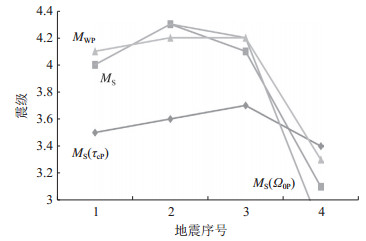图 7 震级检验对比 Fig.7 The magnitude comparison of the inspection earthquake

5 结论

 陈学忠, 李艳娥. 2006年7月4日河北文安MS 5.1地震前震中周围地区小震视应力随时间的变化[J]. 中国地震, 2007, 23(4): 327-336. DOI:10.3969/j.issn.1001-4683.2007.04.001 金星, 张红才, 李军, 等. 地震预警震级确定方法研究[J]. 地震学报, 2012, 34(5): 593-610. DOI:10.3969/j.issn.0253-3782.2012.05.002 翟璐媛, 杨陈. 使用P波快速测定国家台网大震标准震级[J]. 震害防御技术, 2015, 10(2): 378-387. Ellsworth W L, Beroza G C. Seismic evidence for an earthquake nucleation phase[J]. Science, 1995, 268(5 212): 851-855. Iio Y. Slow initial phase of the P-wave velocity pulse generated by microearthquakes[J]. Geophysical Research Letters, 1992, 19(5): 477-480. DOI:10.1029/92GL00179 Iio Y. Observations of the slow initial phase generated by microearthquakes:Implications for earthquake nucleation and propagation[J]. Geophysical Research, 1995, 100(B8): 15333-15349. DOI:10.1029/95JB01150 Kanamori H. The energy release in great earthquakes[J]. J Geophys Res, 1977, 82(20): 2981-2987. DOI:10.1029/JB082i020p02981 Kanamori H. Real-time seismology and earthquake damage mitigation[J]. Annual Review of Earthquake and Planetary Sciences, 2005, 33: 195-214. DOI:10.1146/annurev.earth.33.092203.122626 Nakatani M, Kaneshima S, Fukao Y. Size dependent microearthquake initiation inferred from high-gain and low noise observation at Nikko district, Japan[J]. J Geophys Res, 2000, 105(B12): 28095-28109. DOI:10.1029/2000JB900255 Tsuboi S. Application of MWP to Tsunami Earthquake[J]. Geophysical Research Letters, 2000, 27(19): 3105-3108. DOI:10.1029/2000GL011735 Umeda Y. High-amplitude seismic waves radiated from the bright spot of an earthquake[J]. Tectonophysics, 1990, 175(1/2/3): 81-92.